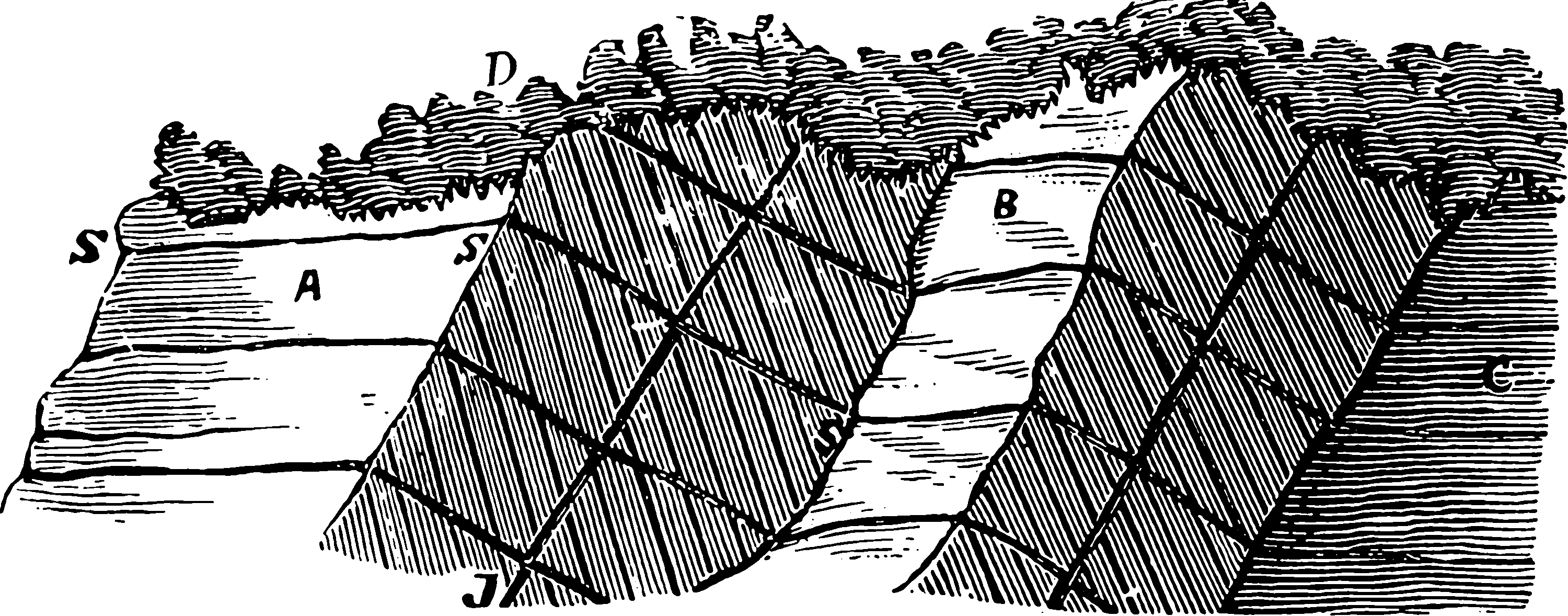# Gaussian processes on lattices$$\renewcommand{\var}{\operatorname{Var}} \renewcommand{\dd}{\mathrm{d}} \renewcommand{\pd}{\partial} \renewcommand{\vv}{\boldsymbol{#1}} \renewcommand{\mm}{\mathrm{#1}} \renewcommand{\mmm}{\mathrm{#1}} \renewcommand{\cc}{\mathcal{#1}} \renewcommand{\oo}{\operatorname{#1}} \renewcommand{\gvn}{\mid} \renewcommand{\II}{\mathbb{I}\{#1\}} \renewcommand{\inner}{\langle #1,#2\rangle} \renewcommand{\Inner}{\left\langle #1,#2\right\rangle} \renewcommand{\norm}{\| #1\|} \renewcommand{\Norm}{\|\langle #1\right\|} \renewcommand{\argmax}{\operatorname{arg max}} \renewcommand{\argmin}{\operatorname{arg min}} \renewcommand{\omp}{\mathop{\mathrm{OMP}}}$$

Gaussian Processes with a stationary kernel are faster if you are working on a grid of points. I have not used this trick, but I understand it involves various simplifications arising from the structure of Gram matrices which end up being Kronecker products of Toeplitz matrices under lexical ordering of the input points, which is long words to describe a fairly simple thing. The keyword to highlight this is Kronecker inference in the ML literature . Another keyword is circulant embedding, the approximation of a Toeplitz matrix by a circulant matrix, which apparently enables one to leverage fast Fourier transforms to calculate some quantities of interest, and some other nice linear algebra properties besides. That would imply that this method is has its origins in Whittle likelihoods , if I am not mistaken.

Lattice GP methods complements, perhaps, the trick of filtering Gaussian processes, which can also exploit structure lattice inputs, although the setup is different between these.

TBC.

## In regressionOK, so what does this allow us to do with posterior inference in GPs? Apparently quite a lot. The KISS-GP method presumably leverages something similar. So do Saatçi (2012) and Flaxman et al. (2015).

Flaxman et al. (2015) deals with the lattice structure trick in a non-Gaussian likelihood setting using Laplace approximation.

TBC

### No comments yet. Why not leave one?

GitHub-flavored Markdown & a sane subset of HTML is supported.